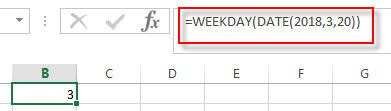# Excel WEEKDAY Function

This post will guide you how to use Excel WEEKDAY function with syntax and examples in Microsoft excel.

### Description

The Excel WEEKDAY function returns a integer value representing the day fo the week for a given Excel date and the value is range from 1 to 7. so you can use this function to get the weekday number for a supplied date. and it will returns 1 for Sunday and returns 7 for Saturday. you also can use the WEEKDAY function to combine with other formulas or functions.

The WEEKDAY function is a build-in function in Microsoft Excel and it is categorized as a DATE and TIME Function.

The WEEKDAY function is available in Excel 2016, Excel 2013, Excel 2010, Excel 2007, Excel 2003, Excel XP, Excel 2000, Excel 2011 for Mac.

### Syntax

The syntax of the WEEKDAY function is as below:

`=WEEKDAY (serial_number,[return_type])`

Where the WEEKDAY function arguments is:

• Serial_number –This is a required argument. An Excel date that you want to get the weekday of. the dates should be typed by the DATE function to make sure it is a valid Excel date. For example, use DATE(2018,3,20) to pass into the WEEKDAY function and then it will return the weekday of this date.
• return_type – This is an optional argument. A number that determines the type of return value.
 Return_type Number returned 1 or omitted Numbers 1 (Sunday) through 7 (Saturday). Behaves like previous versions of Microsoft Excel. 2 Numbers 1 (Monday) through 7 (Sunday). 3 Numbers 0 (Monday) through 6 (Sunday). 11 Numbers 1 (Monday) through 7 (Sunday). 12 Numbers 1 (Tuesday) through 7 (Monday). 13 Numbers 1 (Wednesday) through 7 (Tuesday). 14 Numbers 1 (Thursday) through 7 (Wednesday). 15 Numbers 1 (Friday) through 7 (Thursday). 16 Numbers 1 (Saturday) through 7 (Friday). 17 Numbers 1 (Sunday) through 7 (Saturday).

Note:

• If serial_number is out of range for the current date base value, a #NUM! error is returned.
• If return_type is out of the range specified in the table above, a #NUM! error is returned.
• A serial date is how the Microsoft Excel stores dates and it represents the number of days since 1900-01-01, so the January 1, 1900 date is serial number 1 by default.

### Excel WEEKDAY Function Examples

The below examples will show you how to use Excel WEEKDAY Function to return a weekday of a date.

1# get day of the week with the default return type 1, enter the following formula in Cell B1.

=WEEKDAY(DATE(2018,3,20))it means that the weekday of that date is Tuesday.

### Related Functions

• Excel WEEKNUM function
The Excel WEEKNUM function returns the week number of a specific date, and the returned value is ranging from 1 to 53.The syntax of the WEEKNUM function is as below:=WEEKNUM (serial_number,[return_type])…
• Excel DAY function
The Excel DAY function returns a day of a date (from 1 to 31).The DAY function is a build-in function in Microsoft Excel and it is categorized as a DATE and TIME Function.The syntax of the DAY function is as below:= DAY (date_value)…
• Excel EDATE function
TThe Excel EDATE function returns the serial number that represents the date that is a specified number of months before or after a specified date.The syntax of the EDATE function is as below:=EDATE (start_date, months)…
• Excel DATE function
The Excel DATE function returns the serial number for a date.The syntax of the DATE function is as below:= DATE (year, month, day)…

### More Excel WEEKDAY Function Examples

• Calculate Thanksgiving Date
You can use a simple arithmetic to calculate the possible date range for the US Thanksgiving, it falls on the fourth Thursday in November. And you can create a formula based on the DATE function and the WEEKDAY function.…
• Convert Date to Day of Week in Excel (get day name from date )
If you want to get the day name from a date in excel, you can use the TEXT function with a specified format code like “ddd” or “dddd”..…
• Get the First Monday of a Given Year
To calculate the first Monday of the year or given any date, you can create a new complex formula based on the DATE function, the YEAR function and the WEEKDAY function……
• Converting Week Number to Date
You need to create a formula based on the MAX function, the DATE function and the WEEKDAY function to convert week number to a date in Excel.…